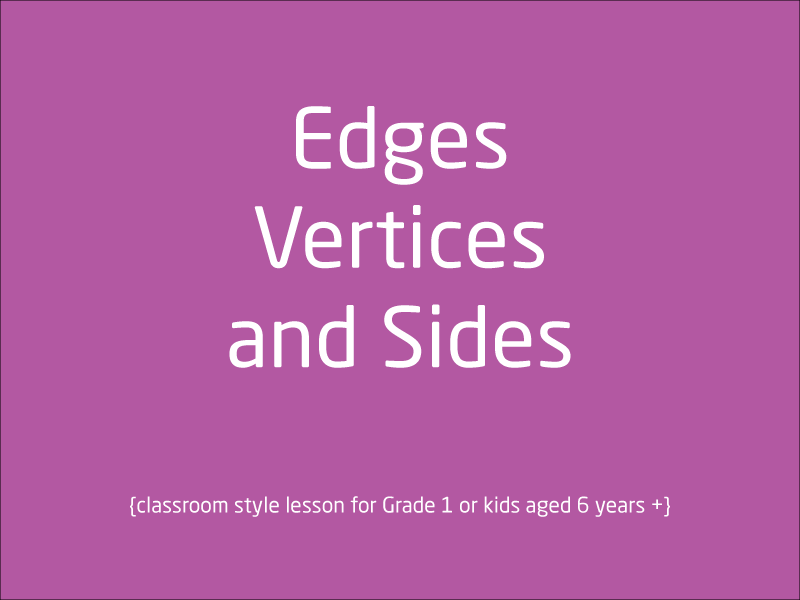# Free video lessons for Foundation to Grade 8 students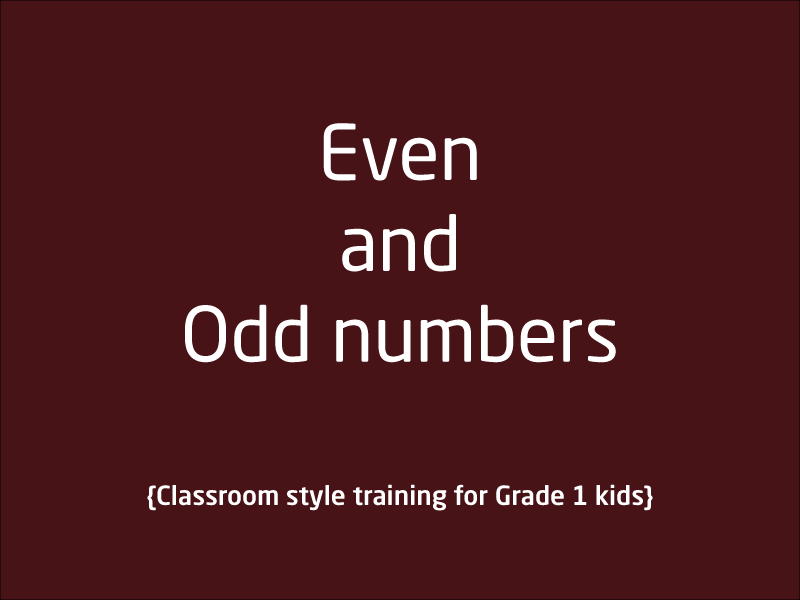### Even and Odd Numbers

What are even numbers? What are odd numbers? This lesson just explains the concepts of Odd and Even numbers. Lesson itself does not tell if Zero is a even or Odd number but as an e...

1 videos
Basic difficulty
0 0 0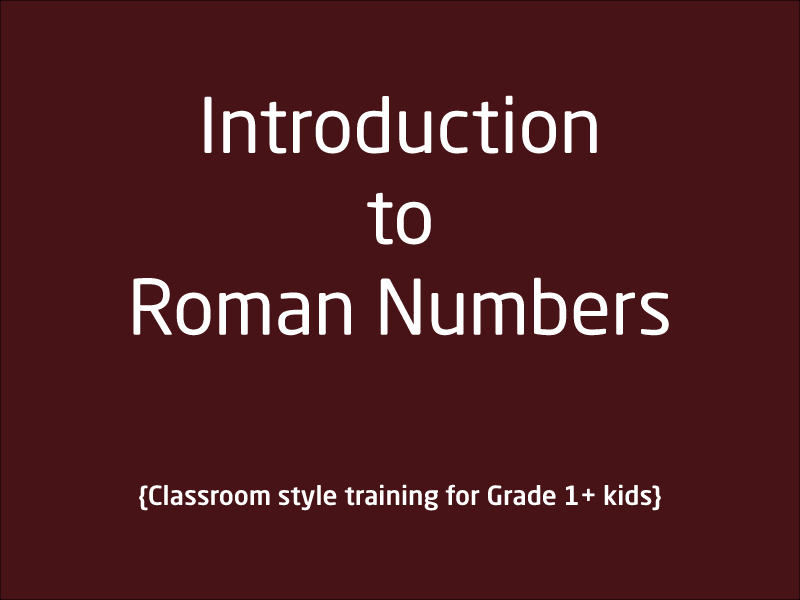### Roman Numbers

This lesson talks about the roman representation of whole/decimal numbers in Maths. Roman numerals are still used in many places. This is a classroom-style lesson and tutor use whi...

1 videos
Intermediate difficulty
0 0 0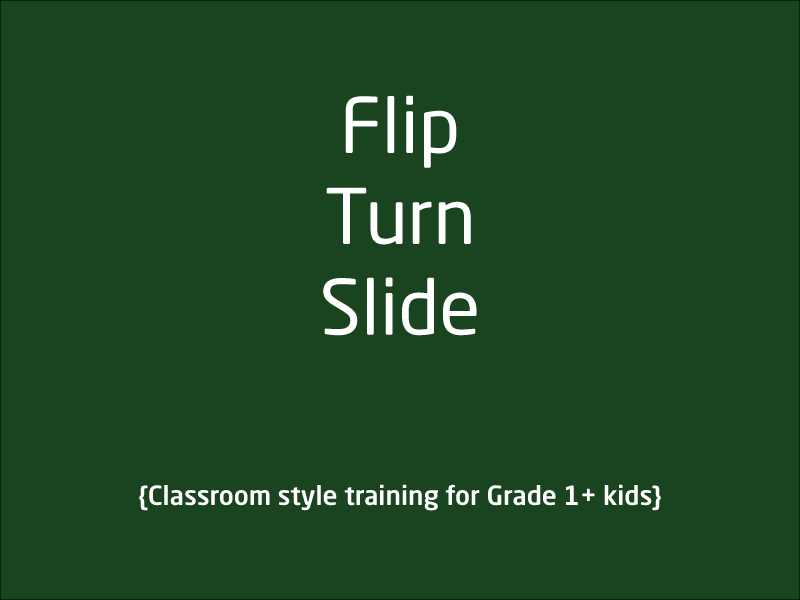### Flip Turn and Slide

In this lesson, tutor use interactive approach to explain fundamentals behind flip, turn and slide. This is a classroom-style lesson and tutor use whiteboard and pen excessively in...

1 videos
Basic difficulty
0 0 0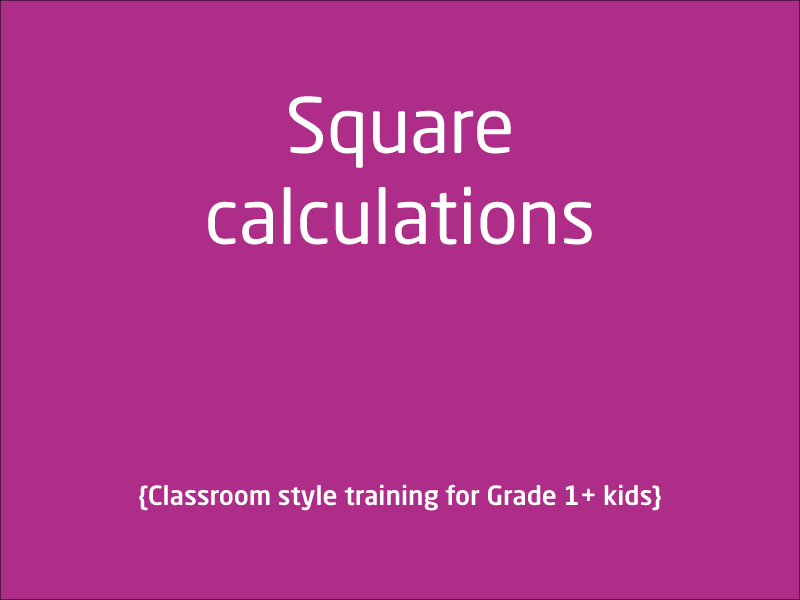### What is Square in Maths?

This lesson is for Grade 1+ kids or kids aged 6 years plus. In this lesson, tutor explain what exactly is a square and how exactly do we calculate the square of a number. Tutor pro...

1 videos
Basic difficulty
0 0 0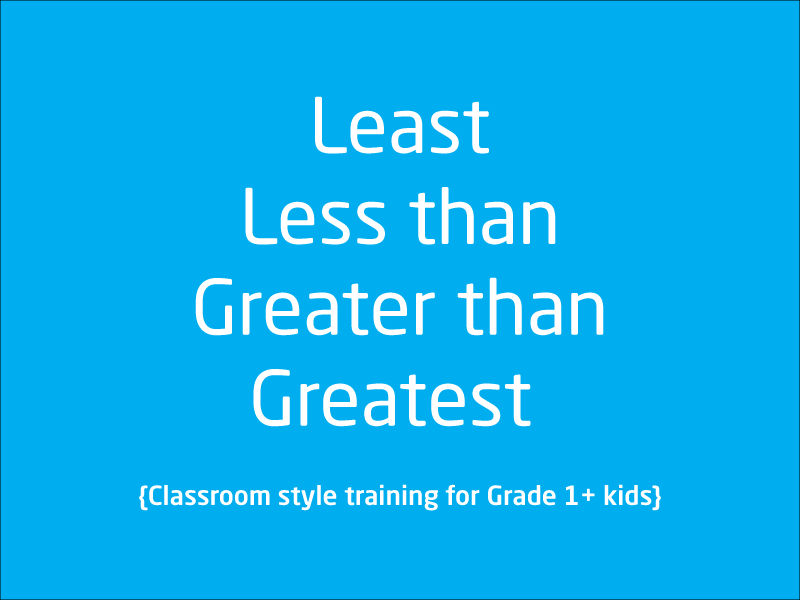### What is Least, Less than, Greater, Greater than

This is a classroom lesson using whiteboard that explains and introduce kids to five terms in mathsLeastLess thanGreaterGreater thanEquals ...

1 videos
Basic difficulty
0 0 0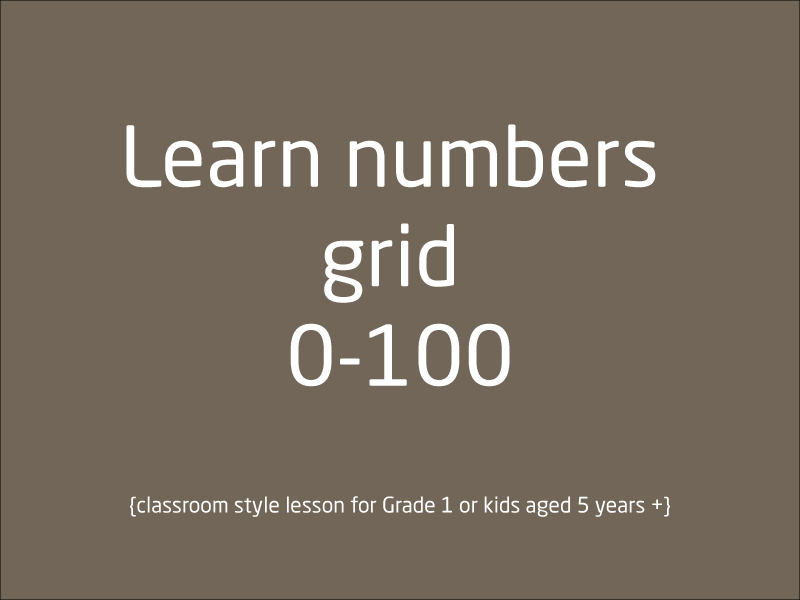### Count from 0 to 100

This classroom is made possible by our household tutor Jas, He teaches kids how to create numbers grid from 0 to 100 so it becomes easy to learn number beyond 0 to 9. There is a ba...

1 videos
Basic difficulty
0 0 0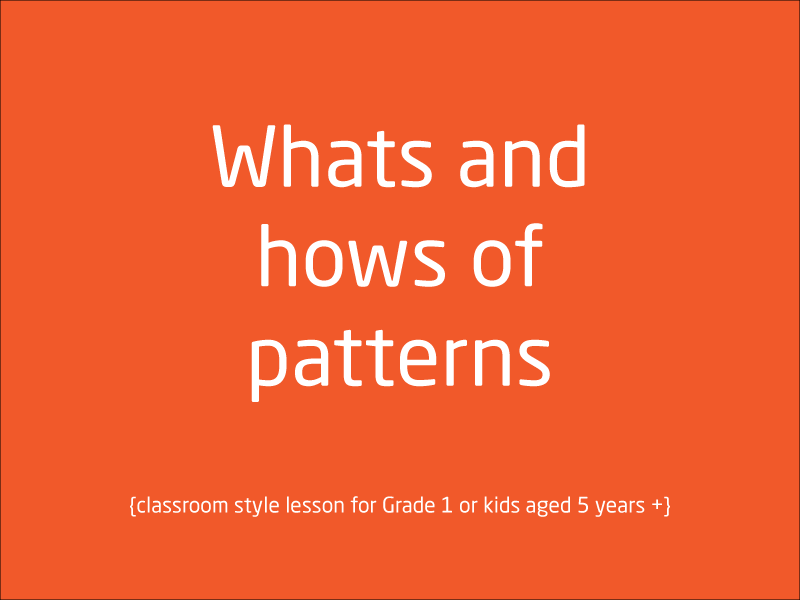This video lesson introduces patterns to little kids. So many examples are shown. Tutor use whiteboard, to explain what patterns are and how to identify them.

1 videos
Basic difficulty
0 0 0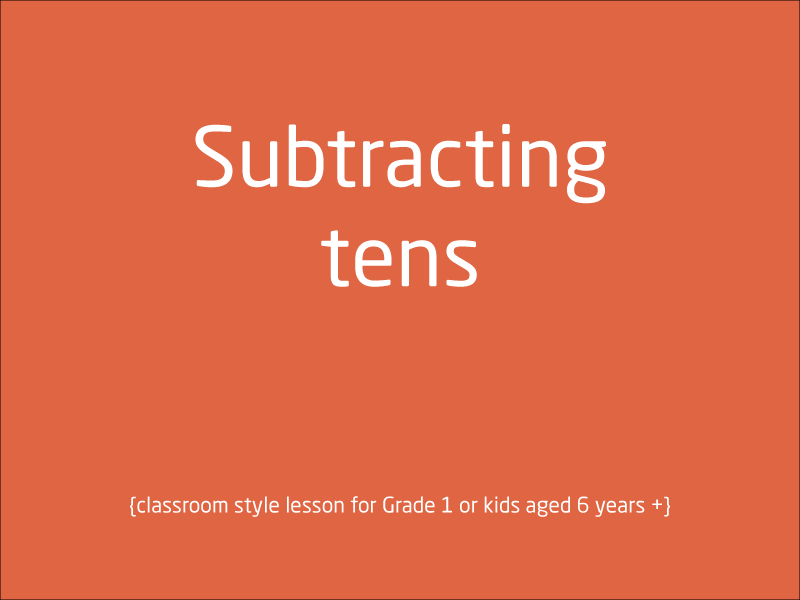### Subtracting numbers that are multiple of tens

Hi Kids, Patience is the key when you take or try to understand this lesson. This could be one big jump from what you have already learnt in the past. We will be subtracting more c...

1 videos
Basic difficulty
0 0 0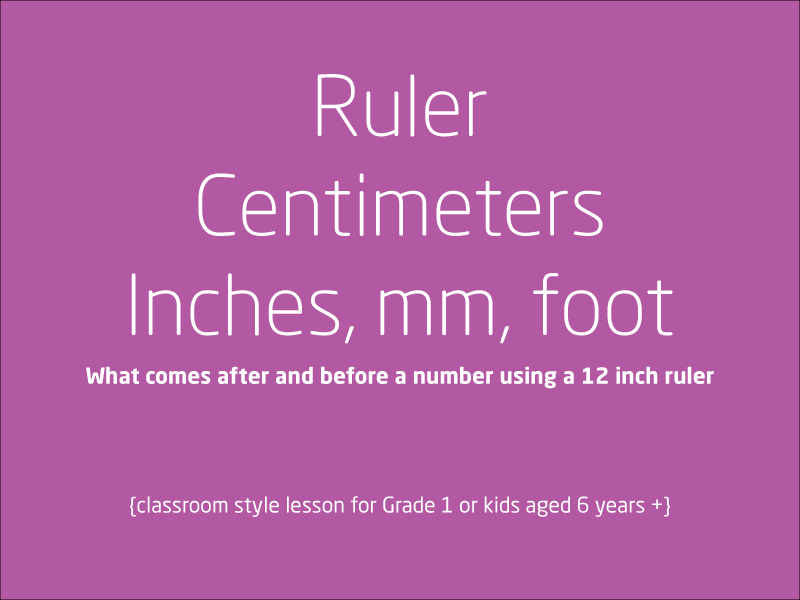### Centimeters, Inches, Foot and millimeters

This lesson has a lot of stuff in it. A 10 minute plus session for kids who wish to know ins and outs of a ruler and how to use it. It also explains length units such as centimeter...

1 videos
Basic difficulty